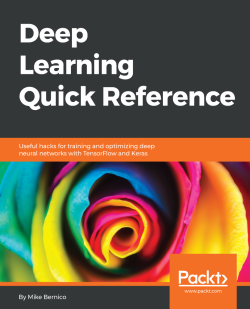# Deep Learning Quick Reference

By Mike Bernico
• FREE Subscription Access now
• \$12.99 eBook + Subscription
What do you get with a Packt Subscription?

• Constantly updated with 100+ new titles each month
• Breadth and depth in over 1,000+ technologies

Deep learning has become an essential necessity to enter the world of artificial intelligence. With this book deep learning techniques will become more accessible, practical, and relevant to practicing data scientists. It moves deep learning from academia to the real world through practical examples.

You will learn how Tensor Board is used to monitor the training of deep neural networks and solve binary classification problems using deep learning. Readers will then learn to optimize hyperparameters in their deep learning models. The book then takes the readers through the practical implementation of training CNN's, RNN's, and LSTM's with word embeddings and seq2seq models from scratch. Later the book explores advanced topics such as Deep Q Network to solve an autonomous agent problem and how to use two adversarial networks to generate artificial images that appear real. For implementation purposes, we look at popular Python-based deep learning frameworks such as Keras and Tensorflow, Each chapter provides best practices and safe choices to help readers make the right decision while training deep neural networks.

By the end of this book, you will be able to solve real-world problems quickly with deep neural networks.

Publication date:
March 2018
Publisher
Packt
Pages
272
ISBN
9781788837996

# The Building Blocks of Deep Learning

Welcome to Deep Learning Quick Reference! In this book, I am going to attempt to make deep learning techniques more accessible, practical, and consumable to data scientists, machine learning engineers, and software engineers who need to solve problems with deep learning. If you want to train your own deep neural network and you're stuck somewhere, there is a good chance this guide will help.

This book is hands on and is intended to be a practical guide that can help you solve your problems fast. It is primarily intended for experienced machine learning engineers and data scientists who need to use deep learning to solve a problem. Aside from this chapter, which provides some of the terminology, frameworks, and background that we will need to get started, it's not meant to be read in order. Each chapter contains a practical example, complete with code and a few best practices and safe choices. We expect you to flip to the chapter you need and get started.

This book won't go deeply into the theory of deep learning and neural networks. There are many wonderful books that can provide that background, and I highly recommend that you read at least one of them (maybe a bibliography or just recommendations). We hope to provide just enough theory and mathematical intuition to get you started.

We will cover the following topics in this chapter:

• Deep neural network architectures
• Optimization algorithms for deep learning
• Deep learning frameworks
• Building datasets for deep learning

# The deep neural network architectures

The deep neural network architectures can vary greatly in structure depending on the network's application, but they all have some basic components. In this section, we will talk briefly about those components.

In this book, I'll define a deep neural network as a network with more than a single hidden layer. Beyond that we won't attempt to limit the membership to the Deep Learning Club. As such, our networks might have less than 100 neurons, or possibly millions. We might use special layers of neurons, including convolutions and recurrent layers, but we will refer to all of these as neurons nonetheless.

# Neurons

A neuron is the atomic unit of a neural network. This is sometimes inspired by biology; however, that's a topic for a different book. Neurons are typically arranged into layers. In this book, if I'm referring to a specific neuron, I'll use the notationwhere l is the layer the neuron is in and k is the neuron number. As we will be using programming languages that observe 0th notation, my notation will also be 0th based.

At their core, most neurons are composed of two functions that work together: a linear function and an activation function. Let us take a high-level look at those two components.

# The neuron linear function

The first component of the neuron is a linear function whose output is the sum of the inputs, each multiplied by a coefficient. This function is really more or less a linear regression. These coefficients are typically referred to as weights in neural network speak. For example, given some neuron with the input features of x1, x2, and x3, and output z, this linear component or the neuron linear function would simply be:Whereare weights or coefficients that we will need to learn given the data and b is a bias term.

# Neuron activation functions

The second function of the neuron is the activation function, which is tasked with introducing a nonlinearity between neurons. A commonly used activation is the sigmoid activation, which you may be familiar with from logistic regression. It squeezes the output of the neuron into an output space where very large values of z are driven to 1 and very small values of z are driven to 0.

The sigmoid function looks like this: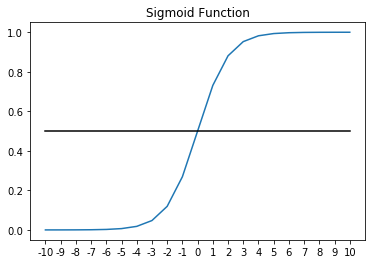It turns out that the activation function is very important for intermediate neurons. Without it one could prove that a stack of neurons with linear activation's (which is really no activation, or more formally an activation function where z=z) is really just a single linear function.

A single linear function is undesirable in this case because there are many scenarios where our network may be under specified for the problem at hand. That is to say that the network can't model the data well because of non-linear relationships present in the data between the input features and target variable (what we're predicting).

The canonical example of a function that cannot be modeled with a linear function is the exclusive OR function, which is shown in the following figure: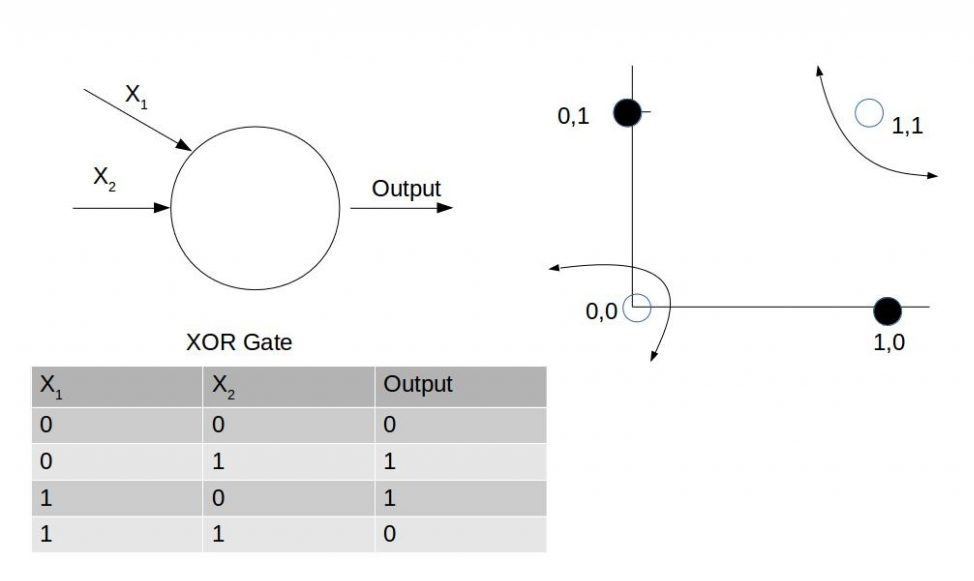Other common activation functions are the tanh function and the ReLu or Rectilinear Activation.

The hyperbolic tangent or the tanh function looks like this:>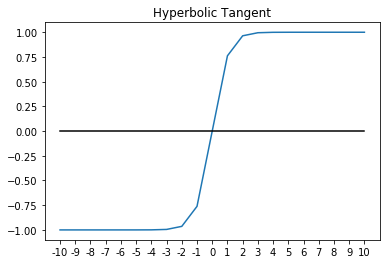The tanh usually works better than sigmoid for intermediate layers. As you can probably see, the output of tanh will be between [-1, 1], whereas the output of sigmoid is [0, 1]. This additional width provides some resilience from a phenomenon known as the vanishing/exploding gradient problem, which we will cover in more detail later. For now, it's enough to know that the vanishing gradient problem can cause networks to converge very slowly in the early layers, if at all. Because of that, networks using tanh will tend to converge somewhat faster than networks that use sigmoid activation. That said, they are still not as fast as ReLu.

ReLu, or Rectilinear Activation, is defined simply as:It's a safe bet and we will use it most of the time throughout this book. Not only is ReLu easy to compute and differentiate, it's also resilient against the vanishing gradient problem. The only drawback to ReLu is that it's first derivative is undefined at exactly 0. Variants including leaky ReLu, are computationally harder, but more robust against this issue.

For completeness, here's a somewhat obvious graph of ReLu: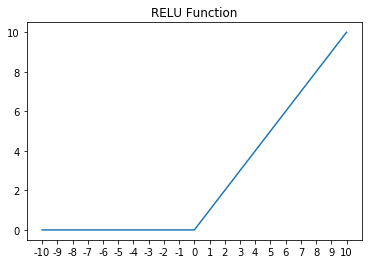# The loss and cost functions in deep learning

Every machine learning model really starts with a cost function. Simply, a cost function allows you to measure how well your model is fitting the training data. In this book, we will define the loss function as the correctness of fit for a single observation within the training set. The cost function will then most often be an average of the loss across the training set. We will revisit loss functions later when we introduce each type of neural network; however, quickly consider the cost function for linear regression as an example:In this case, the loss function would be, which is really the squared error. So then J, our cost function, is really just the mean squared error, or an average of the squared error across the entire dataset. The term 1/2 is added to make some of the calculus cleaner by convention.

# The forward propagation process

Forward propagation is the process by which we attempt to predict our target variable using the features present in a single observation. Imagine we had a two-layer neural network. In the forward propagation process, we would start with the features present within that observationand then multiply those features by their associated coefficients within layer 1 and add a bias term for each neuron. After that, we would send that output to the activation for the neuron. Following that, the output would be sent to the next layer, and so on, until we reach the end of the network where we are left with our network's prediction:

>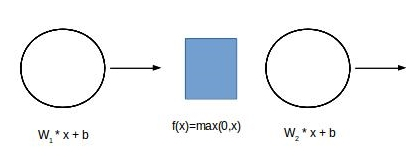# The back propagation function

Once forward propagation is complete, we have the network's prediction for each data point. We also know that data point's actual value. Typically, the prediction is defined aswhile the actual value of the target variable is defined as y.

Once both y andare known, the network's error can be computed using the cost function. Recall that the cost function is the average of the loss function.

In order for learning to occur within the network, the network's error signal must be propagated backwards through the network layers from the last layer to the first. Our goal in back propagation is to propagate this error signal backwards through the network while using it to update the network weights as the signal travels. Mathematically, to do so we need to minimize the cost function by nudging the weights towards values that make the cost function the smallest. This process is called gradient descent.

The gradient is the partial derivative of the error function with respect to each weight within the network. The gradient of each weight can be calculated, layer by layer, using the chain rule and the gradients of the layers above.

Once the gradients of each layer are known, we can use the gradient descent algorithm to minimize the cost function.

The Gradient Descent will repeat this update until the network's error is minimized and the process has converged:The gradient descent algorithm multiples the gradient by a learning rate called alpha and subtracts that value from the current value of each weight. The learning rate is a hyperparameter.

# Stochastic and minibatch gradient descents

The algorithm describe in the previous section assumes a forward and corresponding backwards pass over the entire dataset and as such it's called batch gradient descent.

Another possible way to do gradient descent would be to use a single data point at a time, updating the network weights as we go. This method might help speed up convergence around saddle points where the network might stop converging. Of course, the error estimation of only a single point may not be a very good approximation of the error of the entire dataset.

The best solution to this problem is using mini batch gradient descent, in which we will take some random subset of the data called a mini batch to compute our error and update our network weights. This is almost always the best option. It has the additional benefit of naturally splitting a very large dataset into chunks that are more easily managed in the memory of a machine, or even across machines.

This is an extremely high-level description of one of the most important parts of a neural network, which we believe fits with the practical nature of this book. In practice, most modern frameworks handle these steps for us; however, they are most certainly worth knowing at least theoretically. We encourage the reader to go deeper into forward and backward propagation as time permits.

# Optimization algorithms for deep learning

The gradient descent algorithm is not the only optimization algorithm available to optimize our network weights, however it's the basis for most other algorithms. While understanding every optimization algorithm out there is likely a PhD worth of material, we will devote a few sentences to some of the most practical.

# Using momentum with gradient descent

Using gradient descent with momentum speeds up gradient descent by increasing the speed of learning in directions the gradient has been constant in direction while slowing learning in directions the gradient fluctuates in direction. It allows the velocity of gradient descent to increase.

Momentum works by introducing a velocity term, and using a weighted moving average of that term in the update rule, as follows:Most typicallyis set to 0.9 in the case of momentum, and usually this is not a hyper-parameter that needs to be changed.

# The RMSProp algorithm

RMSProp is another algorithm that can speed up gradient descent by speeding up learning in some directions, and dampening oscillations in other directions, across the multidimensional space that the network weights represent:This has the effect of reducing oscillations more in directions whereis large.

Adam is one of the best performing known optimizer and it's my first choice. It works well across a wide variety of problems. It combines the best parts of both momentum and RMSProp into a single update rule:Whereis some very small number to prevent division by 0.

Adam is often a great choice, and it's a great place to start when you're prototyping, so save yourself some time by starting with Adam.

# Deep learning frameworks

While it's most certainly possible to build and train deep neural networks from scratch using just Python's numpy, that would take a great deal of time and code. It's far more practical, in almost every case, to use a deep learning framework.

Throughout this book we will be using TensorFlow and Keras to make developing deep neural networks much easier and faster.

# What is TensorFlow?

TensorFlow is a library that can be used to quickly build deep neural networks. In TensorFlow, the mathematical operations that we've covered thus far are expressed as nodes. The edges between these nodes are tensors, or multidimensional data arrays. TensorFlow can, given a neural network defined as a graph and a loss function, automatically compute gradients for the network and optimize the graph to minimize the loss function.

TensorFlow was released as an open source project by Google in 2015. Since then it has gained a very large following and enjoys a large user community. While TensorFlow provides APIs in Java, C++, Go, and Python, we will only be covering the Python API. The Python API is used in this book because it's both the most commonly used, and the API most commonly used for the development of new models.

TensorFlow can greatly accelerate computation by performing those calculations on one or more Graphics Processing Units. The acceleration that GPU computation provides has become a necessity in modern deep learning.

# What is Keras?

While building deep neural networks in TensorFlow is far easier than doing it from scratch, TensorFlow is still a very low-level API. Keras is a high-level API that allows us to use TensorFlow (or alternatively Theano or Microsoft's CNTK) to rapidly build deep learning networks.

Models built in Keras and TensorFlow are portable and can be trained or served in native TensorFlow as well. Models constructed in TensorFlow can be loaded into Keras and used there as well.

# Popular alternatives to TensorFlow

There are many other great deep learning frameworks out there. We chose Keras and TensorFlow primarily because of popularity, ease of use, availability for support, and readiness for production deployments. There are undoubtedly other worthy alternatives.

Some of my favorites alternatives to TensorFlow include:

While I do strongly believe that Keras and TensorFlow are the correct choices for this book, I also want to acknowledge these great frameworks and the contributions to the field that each project has made.

# GPU requirements for TensorFlow and Keras

For the remainder of the book, we will be using Keras and TensorFlow. Most of the examples we will be exploring require a GPU for acceleration. Most modern deep learning frameworks, including TensorFlow, use GPUs to greatly accelerate the vast amount of calculations required during network training. Without a GPU, the training time of most of the models we discuss will be unreasonably long.

If you don't have a computer with a GPU installed, GPU-based compute instances can be rented by the second from a variety of cloud providers including Amazon's Amazon Web Services and Google's Google Cloud Platform. For the examples in this book, we will be using a p2.xlarge instance in Amazon EC2 running Ubuntu Server 16.04. The p2.xlarge instance provides an Nvidia Tesla K80 GPU with 2,496 CUDA cores, which will make running the models we show in this book much faster than what is achievable on even very high end desktop computers.

# Installing Nvidia CUDA Toolkit and cuDNN

Since you'll likely be using a cloud based solution for your deep learning work, I've included instructions that will get you up and running fast on Ubuntu Linux, which is commonly available across cloud providers. It's also possible to install TensorFlow and Keras on Windows. As of TensorFlow v1.2, TensorFlow unfortunately does not support GPUs on OS X.

Before we can utilize the GPU, the NVidia CUDA Toolkit and cuDNN must be installed. We will be installing CUDA Toolkit 8.0 and cuDNN v6.0, which are recommended for use with TensorFlow v1.4. There is a good chance that a new version will be released before you finish reading this paragraph, so check www.tensorflow.org for the latest required versions.

We will start by installing the build-essential package on Ubuntu, which contains most of what we need to compile C++ programs. The code is given here:

`sudo apt-get updatesudo apt-get install build-essential`

Next, we can download and install CUDA Toolkit. As previously mentioned, we will be installing version 8.0 and it's associated patch. You can find the CUDA Toolkit that is right for you at https://developer.nvidia.com/cuda-zone.

`wget https://developer.nvidia.com/compute/cuda/8.0/Prod2/local_installers/cuda_8.0.61_375.26_linux-runsudo sh cuda_8.0.61_375.26_linux-run # Accept the EULA and choose defaultswget https://developer.nvidia.com/compute/cuda/8.0/Prod2/patches/2/cuda_8.0.61.2_linux-runsudo sh cuda_8.0.61.2_linux-run # Accept the EULA and choose defaults`

The CUDA Toolkit should now be installed in the following path: /usr/local/cuda. You'll need to add a few environment variables so that TensorFlow can find it. You should probably consider adding these environment variables to ~/.bash_profile, so that they're set at every login, as shown in the following code:

`export LD_LIBRARY_PATH="\$LD_LIBRARY_PATH:/usr/local/cuda/lib64"export CUDA_HOME="/usr/local/cuda"`

At this point, you can test that everything is working by executing the following command: nvidia-smi. The output should look similar to this:

`\$nvidia-smi+-----------------------------------------------------------------------------+ | NVIDIA-SMI 375.26 Driver Version: 375.26 | |-------------------------------+----------------------+----------------------+ | GPU Name Persistence-M| Bus-Id Disp.A | Volatile Uncorr. ECC | | Fan Temp Perf Pwr:Usage/Cap| Memory-Usage | GPU-Util Compute M. | |===============================+======================+======================| | 0 Tesla K80 Off | 0000:00:1E.0 Off | 0 | | N/A 41C P0 57W / 149W | 0MiB / 11439MiB | 99% Default | +-------------------------------+----------------------+----------------------+`

Lastly, we need to install cuDNN, which is the NVIDIA CUDA Deep Neural Network library.

First, download cuDNN to your local computer. To do so, you will need to register as a developer in the NVIDIA Developer Network. You can find cuDNN at the cuDNN homepage at https://developer.nvidia.com/cuDNN. Once you have downloaded it to your local computer, you can use scp to move it to your EC2 instance. While exact instructions will vary by cloud provider you can find additional information about connecting to AWS EC2 via SSH/SCP at https://docs.aws.amazon.com/AWSEC2/latest/UserGuide/AccessingInstancesLinux.html.

Once you've moved cuDNN to your EC2 image, you can unpack the file, using the following code:

`tar -xzvf cudnn-8.0-linux-x64-v6.0.tgz`

Finally, copy the unpacked files to their appropriate locations, using the following code:

`sudo cp cuda/include/cudnn.h /usr/local/cuda/include/sudo cp cuda/lib64/* /usr/local/cuda/lib64`
It's unclear to me why CUDA and cuDNN are distributed separately and why cuDNN requires registrations. The overly complicated download process and manual installation of cuDNN is really one of the greatest mysteries in deep learning.

# Installing Python

We will be using virtualenv to create an isolated Python virtual environment. While this isn't strictly necessary, it's an excellent practice. By doing so, we will keep all our Python libraries for this project in a separate isolated environment that won't interfere with the system Python installation. Additionally, virtualenv environments will make it easier to package and deploy our deep neural networks later on.

Let's start by installing Python, pip, and virtualenv, using the aptitude package manager in Ubuntu. The following is the code:

`sudo apt-get install python3-pip python3-dev python-virtualenv`

Now we can create a virtual environment for our work. We will be keeping all our virtual environment files in a folder called ~/deep-learn. You are free to choose any name you wish for this virtual environment. The following code shows how to create a virtual environment:

`virtualenv --no-site-packages -p python3 ~/deep-learn`
If you're an experienced Python developer, you might have noticed that I've set up the environment to default to Python 3.x. That's most certainly not required, and TensorFlow / Keras both support Python 2.7. That said, the author feels a moral obligation to the Python community to support modern versions of Python.

Now that the virtual environment has been created, you can activate it as follows:

`\$source ~/deep-learn/bin/activate(deep-learn)\$ # notice the shell changes to indicate the virtualenv`
At this point, every time you log in you will need to activate the virtual environment you want to work in. If you would like to always enter the virtual environment you just created, you can add the source command to ~/.bash_profile.

Now that we've configured our virtual environment, we can add Python packages as required within it. To start, let's make sure we have the latest version of pip, the Python package manager:

`easy_install -U pip`

Lastly, I recommend installing IPython, which is an interactive Python shell that makes development much easier.

`pip install ipython`

And that's it. Now we're ready to install TensorFlow and Keras.

# Installing TensorFlow and Keras

After everything we've just been through together, you'll be pleased to see how straightforward installing TensorFlow and Keras now is.

The installation of TensorFlow can be done using the following code:

`pip install --upgrade tensorflow-gpu `
Be sure to pip install tensorflow-gpu. If you pip install TensorfFow (without -gpu), you will install the CPU-only version.

Before we install Keras, let's test our TensorFlow installation. To do this, I'll be using some sample code from the TensorfFow website and the IPython interpreter.

Start the IPython interpreter by typing IPython at the bash prompt. Once IPython has started, let's attempt to import TensorFlow. The output would look like the following:

`In : import tensorflow as tfIn : `

If importing TensorFlow results in an error, troubleshoot the steps you have followed so far. Most often when TensorFlow cannot be imported, the CUDA or cuDNN might not be installed correctly.

Now that we've successfully installed TensorFlow, we will run a tiny bit of code in IPython that will verify we can run computations on the GPU:

`a = tf.constant([1.0,</span> 2.0, 3.0, 4.0, 5.0, 6.0], shape=[2, 3], name='a')b = tf.constant([1.0, 2.0, 3.0, 4.0, 5.0, 6.0], shape=[3, 2], name='b')c = tf.matmul(a, b)sess = tf.Session(config=tf.ConfigProto(log_device_placement=True))print(sess.run(c))`

If everything goes as we hope, we will see lots of indications that our GPU is being used. I have included some output here and highlighted the evidence to draw your attention to it. Your output will likely be different based on hardware, but you should see similar evidence the one shown here:

`/job:localhost/replica:0/task:0/device:GPU:0 -> device: 0, name: Tesla K80, pci bus id: 0000:00:1e.0, compute capability: 3.7MatMul: (MatMul): /job:localhost/replica:0/task:0/device:GPU:0: I tensorflow/core/common_runtime/placer.cc:874] MatMul: (MatMul)/job:localhost/replica:0/task:0/device:GPU:0 b: (Const): /job:localhost/replica:0/task:0/device:GPU:0: I tensorflow/core/common_runtime/placer.cc:874] b: (Const)/job:localhost/replica:0/task:0/device:GPU:0 a: (Const): /job:localhost/replica:0/task:0/device:GPU:0: I tensorflow/core/common_runtime/placer.cc:874] a: (Const)/job:localhost/replica:0/task:0/device:GPU:0 [[ 22. 28.] [ 49. 64.]]`

In the preceding output, we can see that tensors a and b, as well as the matrix multiplication operation, were assigned the the GPU. If there was a problem with accessing the GPU, the output might look as follows:

`I tensorflow/core/common_runtime/placer.cc:874] b_1: (Const)/job:localhost/replica:0/task:0/device:CPU:0a_1: (Const): /job:localhost/replica:0/task:0/device:CPU:0I tensorflow/core/common_runtime/placer.cc:874] a_1: (Const)/job:localhost/replica:0/task:0/device:CPU:0`

Here we can see the tensors b_1 and a_1 were assigned to the CPU rather than the GPU. If this happens there is a problem with your installation of TensorFlow, CUDA, or cuDNN.

If you've made it this far, you have a working installation of TensorFlow. The only remaining task is to install Keras.

The installation of Keras can be done with the help of the following code:

`pip install keras`

And that's it! Now we're ready to build deep neural networks in Keras and TensorFlow.

This might be a great time to create a snapshot or even an AMI of your EC2 instance, so that you don't have to go through this installation again.

# Building datasets for deep learning

Compared to other predictive models that you might have used, deep neural networks are very complicated. Consider a network with 100 inputs, two hidden layers with 30 neurons each, and a logistic output layer. That network would have 3,930 learnable parameters as well as the hyperparameters needed for optimization, and that's a very small example. A large convolutional neural network will have hundreds of millions of learnable parameters. All these parameters are what make deep neural networks so amazing at learning structures and patterns. However, this also makes overfitting possible.

# Bias and variance errors in deep learning

You may be familiar with the so-called bias/variance trade-off in typical predictive models. In case you're not, we'll provide a quick reminder here. With traditional predictive models, there is usually some compromise when we try to find an error from bias and an error from variance. So let's see what these two errors are:

• Bias error: Bias error is the error that is introduced by the model. For example, if you attempted to model a non-linear function with a linear model, your model would be under specified and the bias error would be high.
• Variance error: Variance error is the error that is introduced by randomness in the training data. When we fit our training distribution so well that our model no longer generalizes, we have overfit or introduce a variance error.

In most machine learning applications, we seek to find some compromise that minimizes bias error, while introducing as little variance error as possible. I say most because one of the great things about deep neural networks is that, for the most part, bias and variance can be manipulated independently of one another. However, to do so, we will need to be very careful with how we structure our training data.

# The train, val, and test datasets

For the rest of the book, I will be structuring my data into three separate sets that I'll refer to as train, val, and test. These three separate datasets, drawn as random samples from the total dataset will be structured and sized approximately like this.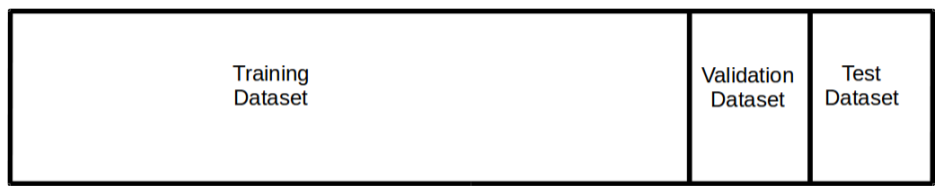The train dataset will be used for training the network, as expected.

The val dataset, or the validation dataset, will be used to find ideal hyperparameters, and to measure overfitting. At the end of an epoch, which is when the network has has the opportunity to observe every data point in the training set, we will make a prediction on the val set. That prediction will be used to watch for overfitting and will help us know when the network has finished training. Using the val set at the end of each epoch like this somewhat differs from the typical usage. For more information on Hold-Out Validation please reference The Elements of Statistical Learning by Hastie and Tibshirani (https://web.stanford.edu/~hastie/ElemStatLearn/).

The test dataset will be used once all training is complete, to accurately measure model performance on a set of data that the network hasn't seen.

It is very important that the val and test data comes from the same datasets. It is less important that the train dataset matches val and test, although that is still ideal. If image augmentation were being used (performing minor modifications to training images in an attempt to amplify the training set size) for example, the training set distribution may no longer match the val set distribution. This is acceptable and network performance can be adequately measured as long as val and test are from the same distribution.

In traditional machine learning applications it's somewhat customary to use 10-20 percent of the available data for val and test. In deep neural networks it's often the case that our data volume is so large that we can adequately measure network performance with much smaller val and test sets. When data volume goes into the 10s of millions of observations, a 98 percent, 1 percent, 1 percent split may be completely appropriate.

# Managing bias and variance in deep neural networks

Now that we've defined how we will structure data and refreshed ourselves on bias and variance, let's consider how we will control bias and variance errors in our deep neural networks.

• High bias: A network with high bias will have a very high error rate when predicting on the training set. The model is not doing well at fitting the data. In order to reduce the bias you will likely need to change the network architecture. You may need to add layers, neurons, or both. It may be that your problem is better solved using a convolutional or recurrent network.

Of course, sometimes a problem is high bias because of a lack of signal or very difficult problem, so be sure to calibrate your expectations on a reasonable rate (I like to start by calibrating on human accuracy).

• High variance: A network with a low bias error is fitting the training data well; however, if the validation error is greater than the test error the network has begun to overfit the training data. The two best ways to reduce variance are by adding data and adding regularization to the network.

Adding data is straightforward but not always possible. Throughout the book, we will cover regularization techniques as they apply. The most common regularization techniques we will talk about are L2 regularization, dropout, and batch normalization.

# K-Fold cross-validation

If you're experienced with machine learning, you may be wondering why I would opt for Hold-Out (train/val/test) validation over K-Fold cross-validation. Training a deep neural network is a very expensive operation, and put very simply, training K of them per set of hyperparameters we'd like to explore is usually not very practical.

We can be somewhat confident that Hold-Out validation does a very good job, given a large enough val and test set. Most of the time, we are hopefully applying deep learning in situations where we have an abundance of data, resulting in an adequate val and test set.

Ultimately, it's up to you. As we will see later, Keras provides a scikit-learn interface that allows Keras models to be integrated into a scikit-learn pipeline. This allows us to perform K-Fold, Stratified K-Fold, and even grid searches with K-Fold. It's both possible and appropriate to sometimes use K-Fold CV in training deep models. That said, for the rest of the book we will focus on the using Hold-Out validation.

# Summary

Hopefully, this chapter served to refresh your memory on deep neural network architectures and optimization algorithms. Because this is a quick reference we didn't go into much detail and I'd encourage the reader to dig deeper into any material here that might be new or unfamiliar.

We talked about the basics of Keras and TensorFlow and why we chose those frameworks for this book. We also talked about the installation and configuration of CUDA, cuDNN, Keras, and TensorFlow.

Lastly, we covered the Hold-Out validation methodology we will use throughout the remainder of the book and why we prefer it to K-Fold CV for most deep neural network applications.

We will be referring back to this chapter quite a bit as we revisit these topics in the chapters to come. In the next chapter, we will start using Keras to solve regression problems, as a first step into building deep neural networks.